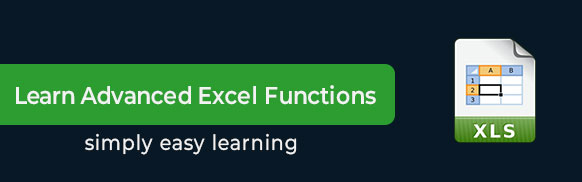This Microsoft Excel tutorial teaches you how to create and use Excel functions. You can use Excel functions to perform various mathematical, statistical, logical calculations. This tutorial takes you step-by-step through the process.

# Audience

This tutorial is intended for people who use Excel but are intimidated by the concept of formulas and functions.

# Prerequisites

This tutorial assumes your familiarity with basic fomulas for calculations in Excel.